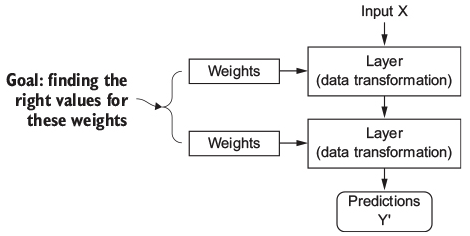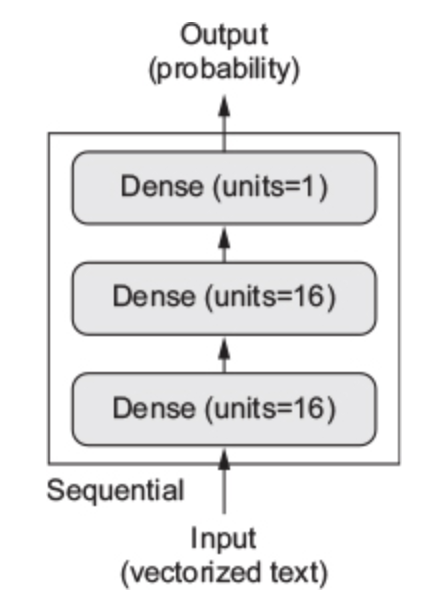A deep-learning model is a directed, acyclic graph of layers.## Layers

Layer is a data-processing component:

• takes one or more tensors as input and outputs one or more tensors.

Stateless or stateful layers:

• Some layers are stateless, but more frequently layers have a state.
• Stateful layers contain parameters that are learned from the data

Types of layers:

• Different layers are appropriate for different tensor formats and different types of data processing.
• For example:
Tensor Type Data Type Data Shape Layer Type Layer Description
2D tensor Vector data (samples, features) layer_dense densely connected layer
3D tensor Sequence data (samples, timesteps, features) layer_lstm recurrent layers
4D tensor Image data (samples, height, width, channels) layer_conv_2d 2D convolution layers

Building deep-learning models in Keras:

• It is done by clipping together compatible layers to form useful data-transformation pipelines.

Layer compatibility:

• Every layer will only accept input tensors of a certain shape and will return output tensors of a certain shape.

• When using Keras, you don’t have to worry about compatibility, because the layers you add to your models are dynamically built to match the shape of the incoming layer.

## Examples

### Fully connected model for MNIST

model <- keras_model_sequential() %>%
layer_dense(units = 512, activation = "relu", input_shape = c(28*28)) %>%
layer_dense(units = 10, activation = "softmax")

### Fully connected model for IMDB

model <- keras_model_sequential() %>%
layer_dense(units = 16, activation = "relu", input_shape = c(10000)) %>%
layer_dense(units = 16, activation = "relu") %>%
layer_dense(units = 1, activation = "sigmoid")

### Fully connected model for Boston House dataset

model <- keras_model_sequential() %>%
layer_dense(units = 64, activation = "relu", input_shape = c(13)) %>%
layer_dense(units = 64, activation = "relu") %>%
layer_dense(units = 1)

## Explaining the code

### The pipe operator

• %>%
• The pipe operator comes from the magrittr package.
• Shorthand for passing the value on its left as the first argument to the function on its right.
model <- keras_model_sequential()
layer_dense(model, units = 512, activation = "relu", input_shape = c(28*28))
layer_dense(model, units = 10, activation = "softmax")
• Besides compactness, the %>% reminds that Keras models are modified in-place.
• You don’t operate on model and then return a new model object.
• Rather, you do something to the model object.

### Linear Stack of Layers

• keras_model_sequential
• Defines a Keral model composed of a linear stack of layers.• Not to be confused with it being a model for sequential data

### Dense layer

layer_dense

• Dense or fully connected layer

Implements the operation: output = activation(dot(input, weight) + bias)

• input: 2D input tensor. Gets flattened if rank > 2.
• weight: 2D weight tensor created by the layer.
• bias: 1D bias tensor created by the layer (use_bias=TRUE).
• dot(input, weight): Dot product between two tensors.
• activation(.): Element-wise activation function.

Most important inputs:

• input_shape: Dimensionality of the input, not including the samples axis.
• Required only for the first layer in a model.
• units: Dimensionality of the output space.
• activation: The name of the activation function. Default to linear.
• relu(x) = max(x,0) is the most commonly used non-linear activation function.

## Some observations

• With Neural Networks, we are able to build models able to capture complex patterns in the data from simple, differentiable operations.
• The importance of the non-linear activation function

Without them each layer would only be able to learn linear transformations of the input data and a deep stack of linear layers would still implement a linear operation. The activation function relu add non-linearity to the model.

## Reference material

This lecture note is based on (Chollet and Allaire 2018).